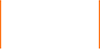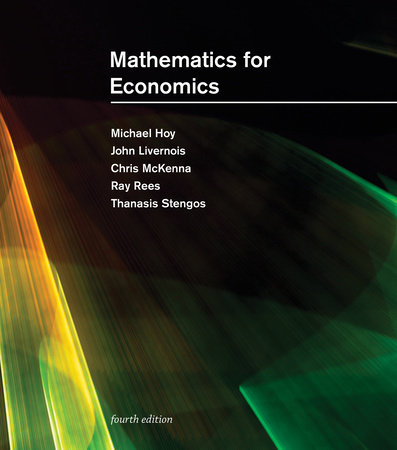# Mathematics for Economics, fourth edition

Best SellerHardcover \$125.00
Mar 29, 2022 | ISBN 9780262046626

#### Hardcover –

• Hardcover \$125.00

Mar 29, 2022 | ISBN 9780262046626

#### Ebook +

• Mar 29, 2022 | ISBN 9780262368797

#### Product Details

Preface xiii
Glossary of Worked Examples xv
Part I Introduction of Fundamentals
Chapter 1
Introduction 3
Chapter 2
Review of the Fundamentals 19
Chapter 3
Sequences, Series, and Limits 75
Part II Univariate Calculus and Optimization
Chapter 4
Continuity of Functions 119
Chapter 5
The Derivative and Differential of Functions of One Variable 145
Chapter 6
Optimization of Functions of One Variable 225
Part III Linear Algebra
Chapter 7
Linear Equations and Vector Spaces 287
Chapter 8
Matrices 333
Chapter 9
Determinants and the Inverse Matrix 365
Chapter 10
Further Topics in Linear Algebra 407
Part IV Multivariate Calculus
Chapter 11
Calculus for Functions of n Variables 443
Chapter 12
Optimization of Functions of n Variables 519
Chapter 13
Constrained Optimization 551
Chapter 14
Comparative Statics 597
Chapter 15
Nonlinear Programming and the Kuhn-Tucker Conditions 635
Part V Integration and Dynamic Methods
Chapter 16
Integration 681
Chapter 17
An Introduction to Mathematics for Economic Dynamics 731
Chapter 18
Linear, First-Order Difference Equations 743
Chapter 19
Nonlinear, First-Order Difference Equations 767
Chapter 20
Linear, Second-Order Difference Equations 783
Chapter 21
Linear, First-Order Differential Equations 817
Chapter 22
Nonlinear, First-Order Differential Equations 843
Chapter 23
Linear, Second-Order Differential Equations 857
Chapter 23
Simultaneous Systems of Differential and Difference Equations 885
Chapter 25
Optimal Control Theory 949